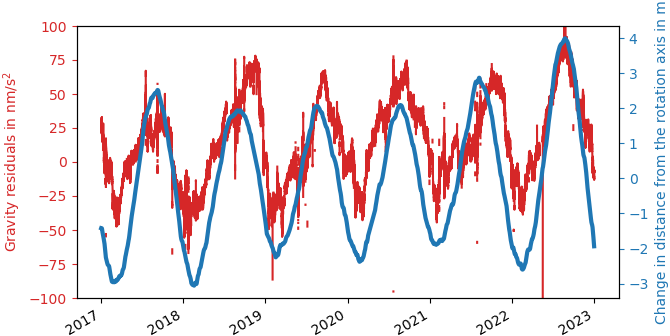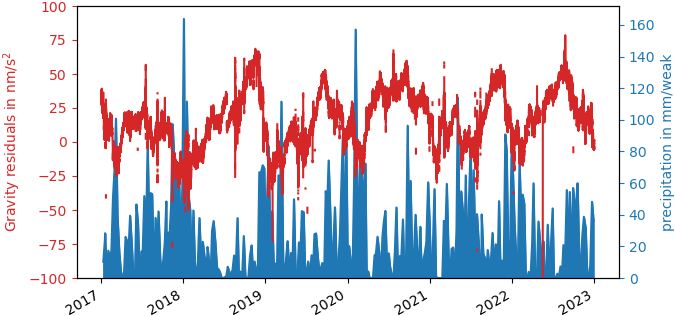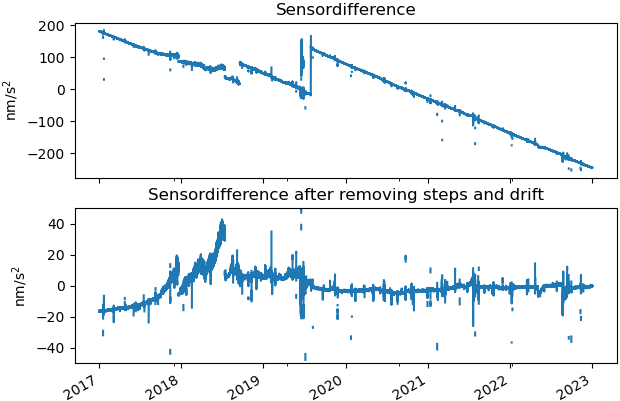# Clara Bützler

Systematic errors of superconducting gravimeters

The value of gravity of about 9.81 m/s2 is not constant, neither in space nor in time. While the biggest spatial differences occur between the equator (9.78 m/s2) and the poles (9.83 m/s2) changes in time are much smaller. The biggest contribution are daily, half-daily and monthly tidal signals originating from the changing gravitational attraction from sun and moon. They reach up to 2 μm/s2. The second-biggest contribution are air pressure changes in the atmosphere above the gravimeter. They can produce gravity changes of about 150 nm/s2. If we correct these two contributions from gravity time series measured with superconducting gravimeters, long term gravity changes at the level of some tens of nm/s2 become visible. Two examples are gravity variations due to polar motion and gravity changes due to local hydrology. As these signals are on the edge of the accuracy of today’s best gravimeters, knowing the error budget of the instruments is very important when studying these signals.Gravity and polar motion. Gravity residuals at BFO are shown together with the variation in distance of the gravimeter to rotation axis of the Earth. The gravity signal was corrected for tides and air pressure variations, and major instrumental disturbances like linear drift and steps.

The axis of rotation is not fixed inside the Earth, but slightly moving. The two main periods of this movement are one year and 430 days. This so-called polar motion influences gravity by two mechanisms. If the pole moves away from us first the centrifugal acceleration increases, which leads to a decrease of gravity. Secondly, the Earth deforms due to the change in centrifugal acceleration, which moves the gravimeter slightly away from the center of mass of the Earth. That again leads to a decrease of gravity. The second effect is about ten times smaller than the first one. However, if we accurately measure this second effect, we know how the Earth deformed due to the change in centrifugal acceleration. By this we could learn more about the viscosity of the mantle. In Figure 1 one can see a time series of gravity measured with the superconducting gravimeter SG056 at the Black Forest observatory (BFO). Additionally, the varying distance of the gravimeter to the rotation axis of the Earth is shown.Gravity and hydrology. The figure shows gravity residuals and weakly amounts of rain at BFO. The gravity signal is the same as in Figure 1 but additionally corrected for polar motion.

Local hydrology also influences gravity. In Figure 2 one can see gravity at BFO together with the monthly amount of rain. It can be seen, that gravity gets smaller in rainy periods. By combining gravity measurements with other measurements, like rainfall and soil moisture, we may learn more about the local hydrology.
Due to their low drift and good long term stability, superconducting gravimeters are used to study long term changes of gravity. They can measure gravity changes in time. However, superconducting gravimeters also show systematical measurement errors. Dual sphere superconducting gravimeters offer one possibility to study them. These are instruments which have two sensing units. That means they are measuring gravity twice inside the instrument. In Figure 3 one can see the difference between the signals of the two sensors of SG056. For a perfect instrument, they should be constant. However, even after removing a linear drift and major steps related to operator interventions, this difference still shows variations at the level of half the amplitude of the gravity residuals themselves. This means we have to be aware of these errors whenever studying long term gravity changes at the ten nm/s2 level.
At GIS and BFO we work on quantifying and characterizing these systematical errors and studying their influence on studies of long term gravity variations related to polar motion or hydrology.Sensor difference of the gravimeter SG056 at BFO. In the top panel the difference between the results of the two measurement units of the gravimeter SG056 at BFO are shown. In the lower panel major steps and drift were removed from the difference.